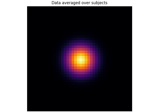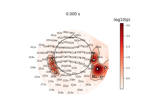# mne.stats.permutation_t_test#

mne.stats.permutation_t_test(X, n_permutations=10000, tail=0, n_jobs=None, seed=None, verbose=None)[source]#

One sample/paired sample permutation test based on a t-statistic.

This function can perform the test on one variable or simultaneously on multiple variables. When applying the test to multiple variables, the “tmax” method is used for adjusting the p-values of each variable for multiple comparisons. Like Bonferroni correction, this method adjusts p-values in a way that controls the family-wise error rate. However, the permutation method will be more powerful than Bonferroni correction when different variables in the test are correlated (see ).

Parameters:
X`array`, shape (n_samples, n_tests)

Samples (observations) by number of tests (variables).

n_permutations`int` | ‘all’

Number of permutations. If n_permutations is ‘all’ all possible permutations are tested. It’s the exact test, that can be untractable when the number of samples is big (e.g. > 20). If n_permutations >= 2**n_samples then the exact test is performed.

tail-1 or 0 or 1 (default = 0)

If tail is 1, the alternative hypothesis is that the mean of the data is greater than 0 (upper tailed test). If tail is 0, the alternative hypothesis is that the mean of the data is different than 0 (two tailed test). If tail is -1, the alternative hypothesis is that the mean of the data is less than 0 (lower tailed test).

n_jobs

The number of jobs to run in parallel. If `-1`, it is set to the number of CPU cores. Requires the `joblib` package. `None` (default) is a marker for ‘unset’ that will be interpreted as `n_jobs=1` (sequential execution) unless the call is performed under a `joblib.parallel_backend()` context manager that sets another value for `n_jobs`.

seed`None` | `int` | instance of `RandomState`

A seed for the NumPy random number generator (RNG). If `None` (default), the seed will be obtained from the operating system (see `RandomState` for details), meaning it will most likely produce different output every time this function or method is run. To achieve reproducible results, pass a value here to explicitly initialize the RNG with a defined state.

verbose

Control verbosity of the logging output. If `None`, use the default verbosity level. See the logging documentation and `mne.verbose()` for details. Should only be passed as a keyword argument.

Returns:
T_obs`array` of shape [n_tests]

T-statistic observed for all variables.

p_values`array` of shape [n_tests]

P-values for all the tests (a.k.a. variables).

H0`array` of shape [n_permutations]

T-statistic obtained by permutations and t-max trick for multiple comparison.

Notes

If `n_permutations >= 2 ** (n_samples - (tail == 0))`, `n_permutations` and `seed` will be ignored since an exact test (full permutation test) will be performed.

References

## Examples using `mne.stats.permutation_t_test`#Statistical inference

Statistical inferencePermutation T-test on sensor data

Permutation T-test on sensor data